# Newton's law of motion Questions and Answers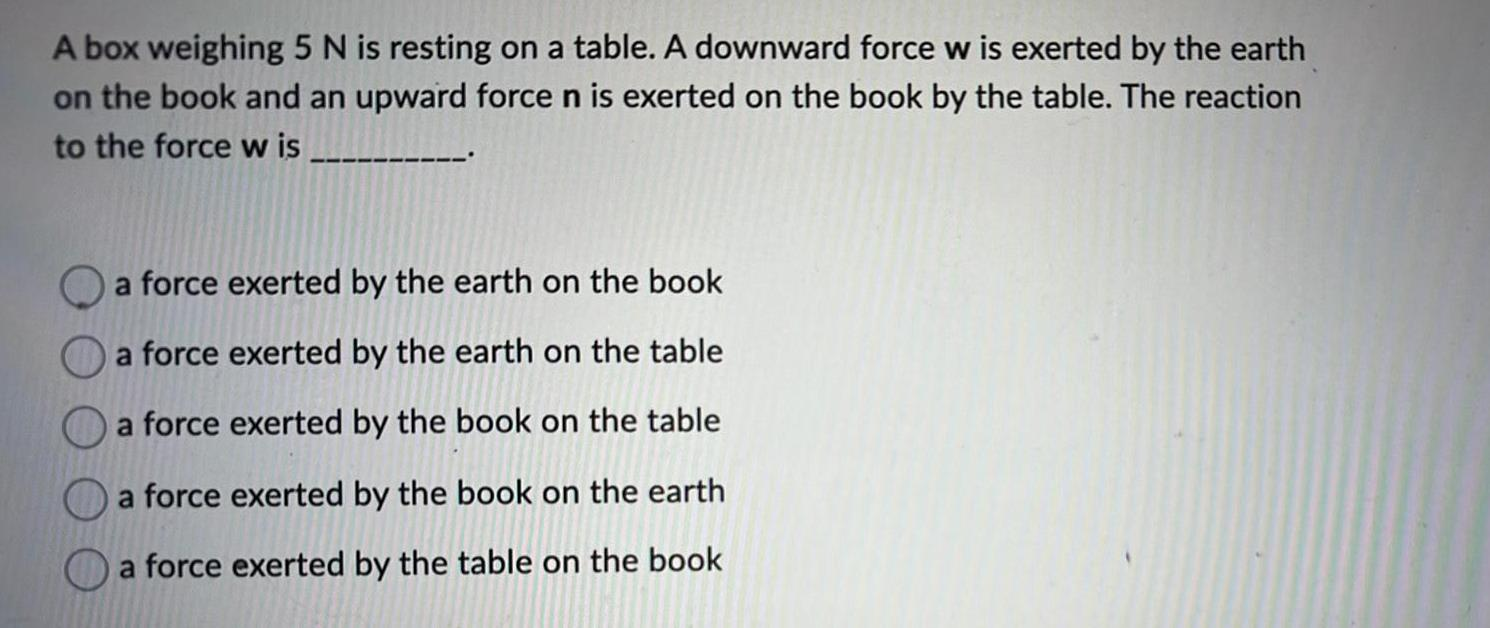Physics
Newton's law of motion
A box weighing 5 N is resting on a table. A downward force w is exerted by the earth on the book and an upward force n is exerted on the book by the table. The reaction to the force w is a force exerted by the earth on the book a force exerted by the earth on the table a force exerted by the book on the table a force exerted by the book on the earth a force exerted by the table on the book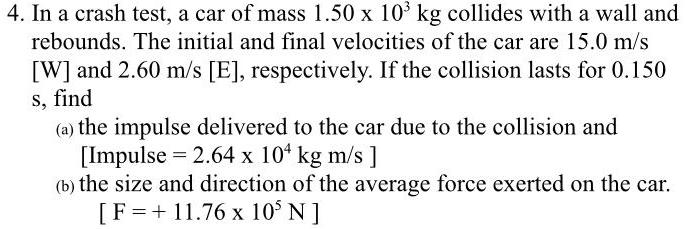Physics
Newton's law of motion
In a crash test, a car of mass 1.50 x 10³ kg collides with a wall and rebounds. The initial and final velocities of the car are 15.0 m/s [W] and 2.60 m/s [E], respectively. If the collision lasts for 0.150 s, find (a) the impulse delivered to the car due to the collision and [Impulse = 2.64 x 104 kg m/s] (b) the size and direction of the average force exerted on the car. [F=+11.76 x 10³ N ]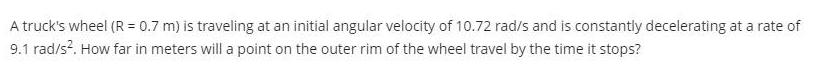Physics
Newton's law of motion
A truck's wheel (R = 0.7 m) is traveling at an initial angular velocity of 10.72 rad/s and is constantly decelerating at a rate of 9.1 rad/s². How far in meters will a point on the outer rim of the wheel travel by the time it stops?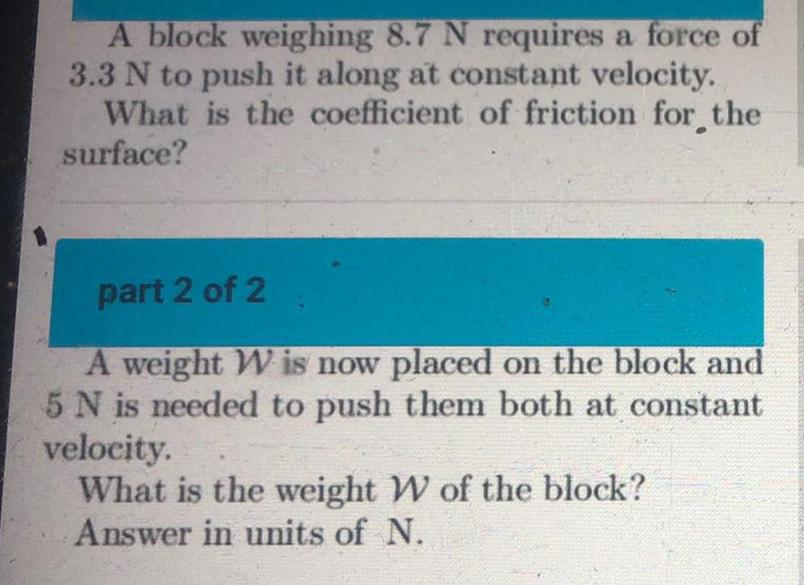Physics
Newton's law of motion
A block weighing 8.7 N requires a force of 3.3 N to push it along at constant velocity. What is the coefficient of friction for the surface?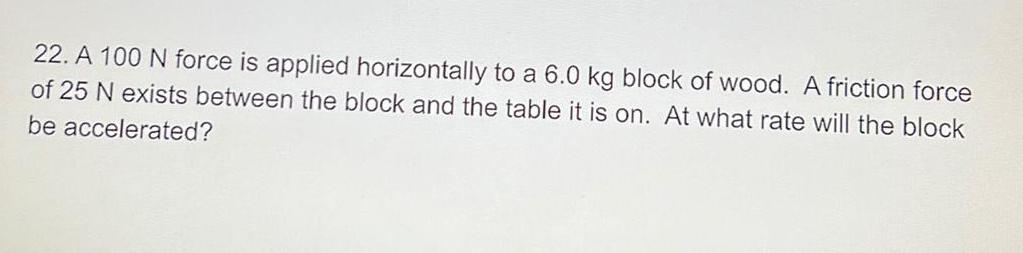Physics
Newton's law of motion
A 100 N force is applied horizontally to a 6.0 kg block of wood. A friction force of 25 N exists between the block and the table it is on. At what rate will the block be accelerated?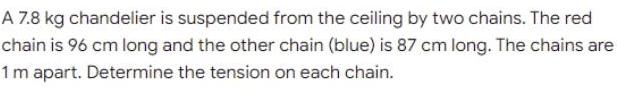Physics
Newton's law of motion
A 7.8 kg chandelier is suspended from the ceiling by two chains. The red chain is 96 cm long and the other chain (blue) is 87 cm long. The chains are 1 m apart. Determine the tension on each chain.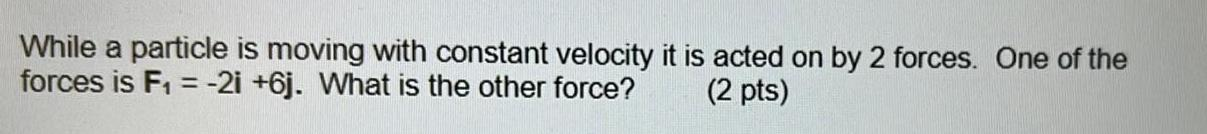Physics
Newton's law of motion
While a particle is moving with constant velocity it is acted on by 2 forces. One of the forces is F₁ = -2i +6j. What is the other force?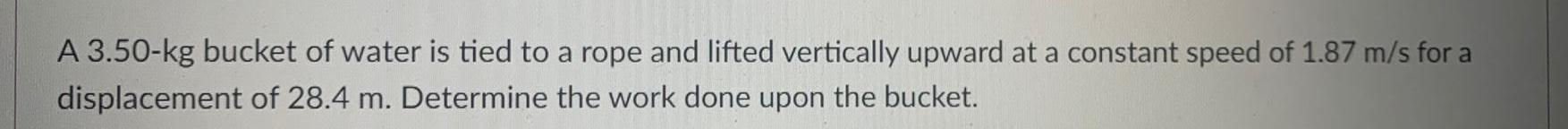Physics
Newton's law of motion
A 3.50-kg bucket of water is tied to a rope and lifted vertically upward at a constant speed of 1.87 m/s for a displacement of 28.4 m. Determine the work done upon the bucket.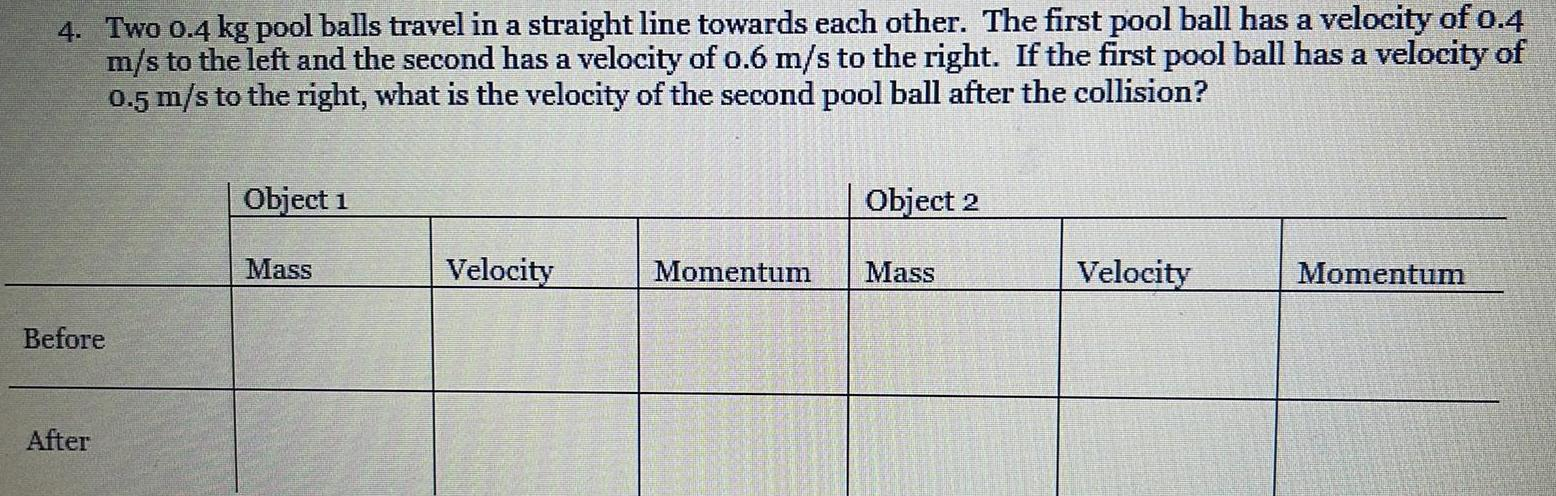Physics
Newton's law of motion
4. Two 0.4 kg pool balls travel in a straight line towards each other. The first pool ball has a velocity of 0.4 m/s to the left and the second has a velocity of 0.6 m/s to the right. If the first pool ball has a velocity of 0.5 m/s to the right, what is the velocity of the second pool ball after the collision? Before After Object 1 Mass Velocity Momentum Object 2 Mass Velocity Momentum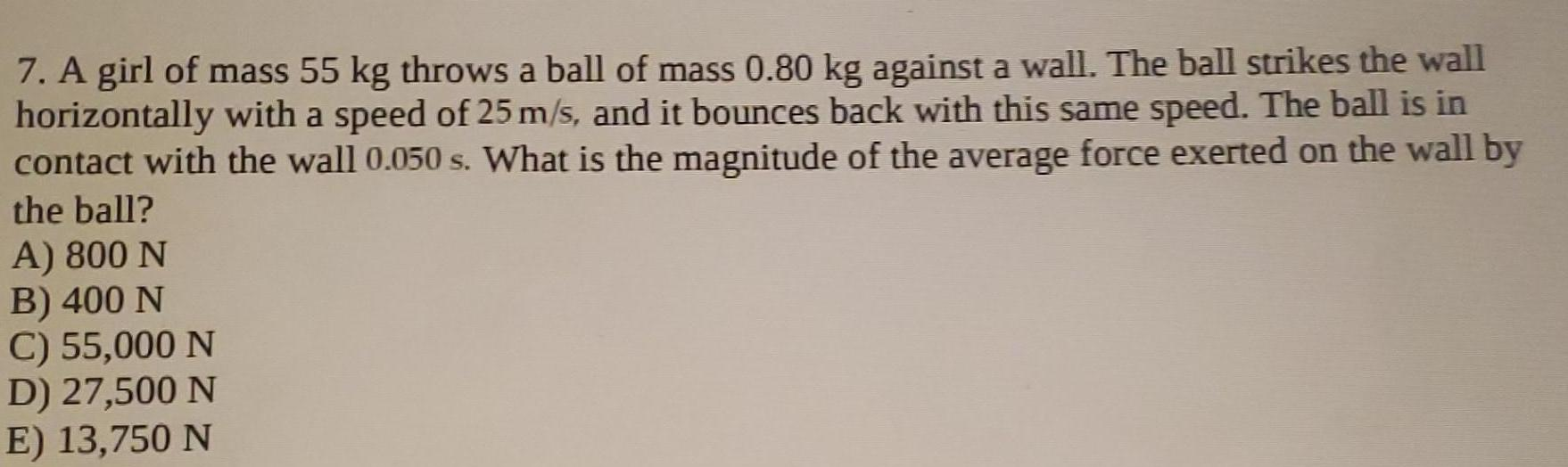Physics
Newton's law of motion
A girl of mass 55 kg throws a ball of mass 0.80 kg against a wall. The ball strikes the wall horizontally with a speed of 25 m/s, and it bounces back with this same speed. The ball is in contact with the wall 0.050 s. What is the magnitude of the average force exerted on the wall by the ball? A) 800 N B) 400 N C) 55,000 N D) 27,500 N E) 13,750 N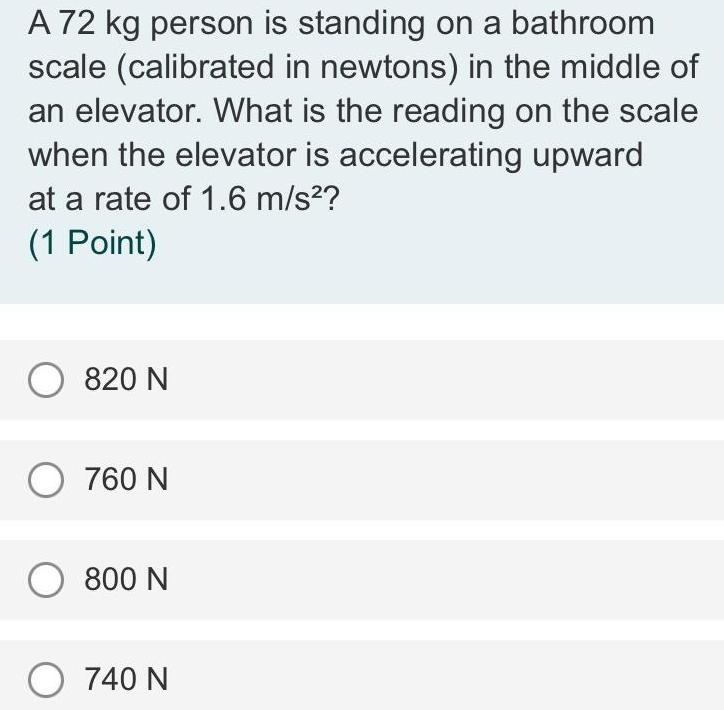Physics
Newton's law of motion
A 72 kg person is standing on a bathroom scale (calibrated in newtons) in the middle of an elevator. What is the reading on the scale when the elevator is accelerating upward at a rate of 1.6 m/s²? 820 N 760 N 800 N 740 N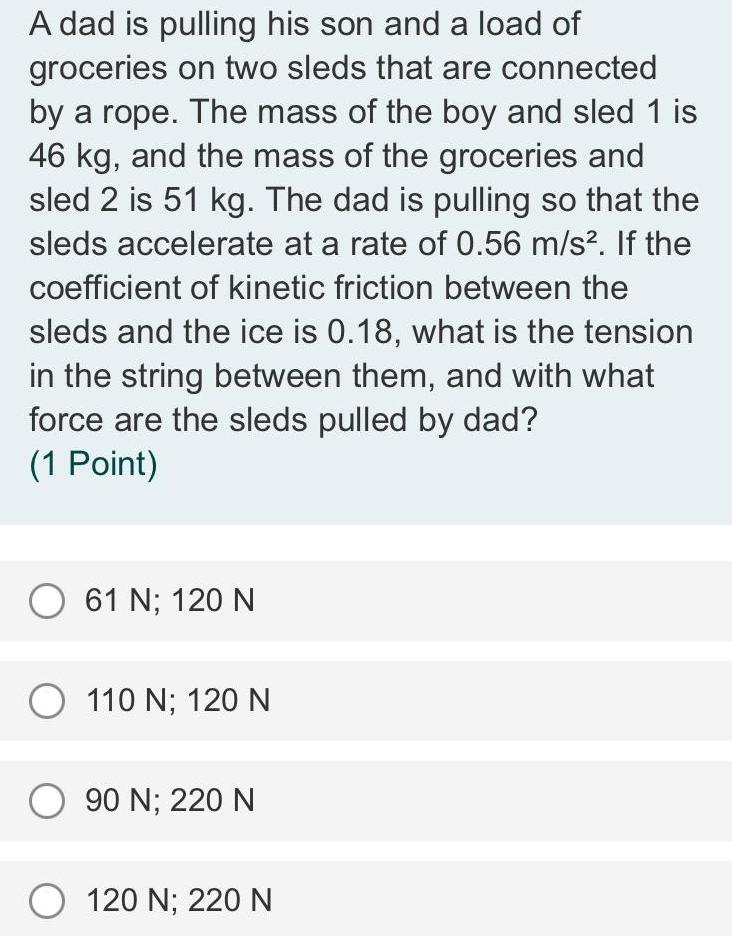Physics
Newton's law of motion
A dad is pulling his son and a load of groceries on two sleds that are connected by a rope. The mass of the boy and sled 1 is 46 kg, and the mass of the groceries and sled 2 is 51 kg. The dad is pulling so that the sleds accelerate at a rate of 0.56 m/s². If the coefficient of kinetic friction between the sleds and the ice is 0.18, what is the tension in the string between them, and with what force are the sleds pulled by dad? (1 Point) 61 N; 120 N 110 N; 120 N 90 N; 220 N 120 N; 220 N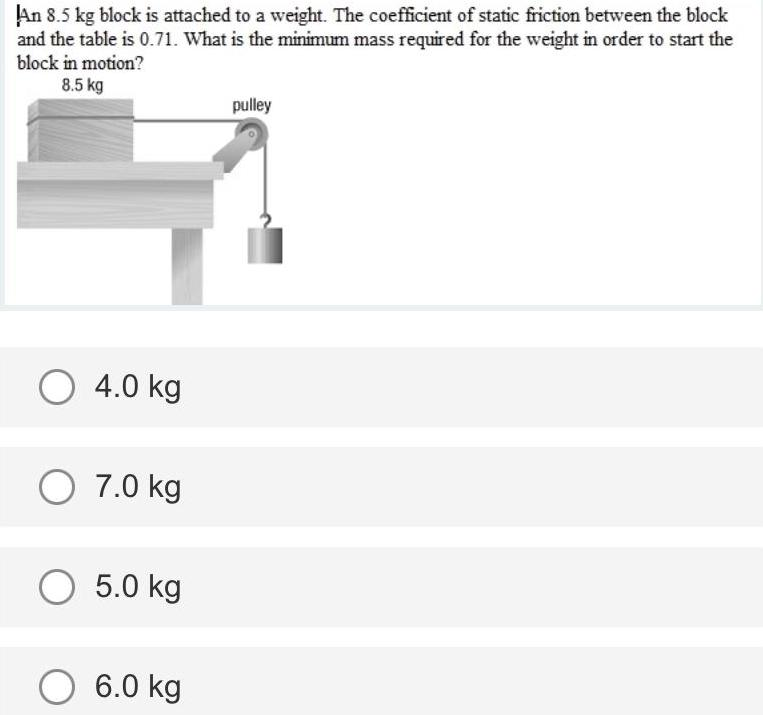Physics
Newton's law of motion
An 8.5 kg block is attached to a weight. The coefficient of static friction between the block and the table is 0.71. What is the minimum mass required for the weight in order to start the block in motion? 4.0 kg 7.0 kg 5.0 kg 6.0 kg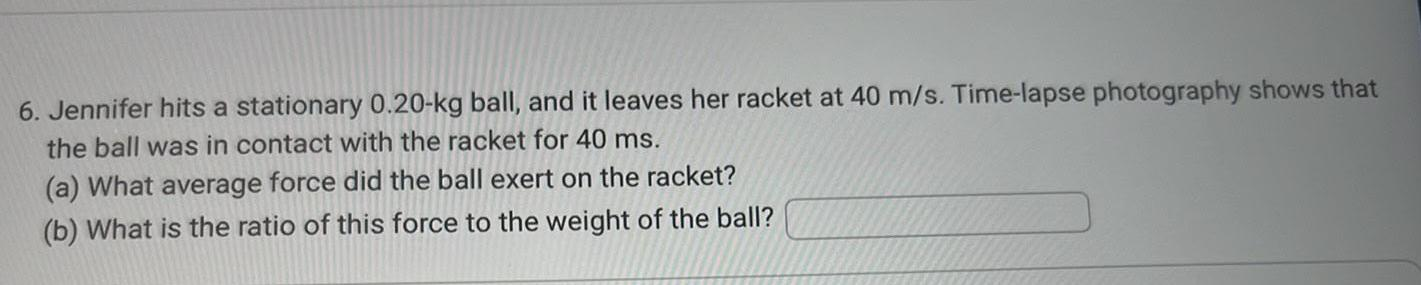Physics
Newton's law of motion
Jennifer hits a stationary 0.20-kg ball, and it leaves her racket at 40 m/s. Time-lapse photography shows that the ball was in contact with the racket for 40 ms. (a) What average force did the ball exert on the racket? (b) What is the ratio of this force to the weight of the ball?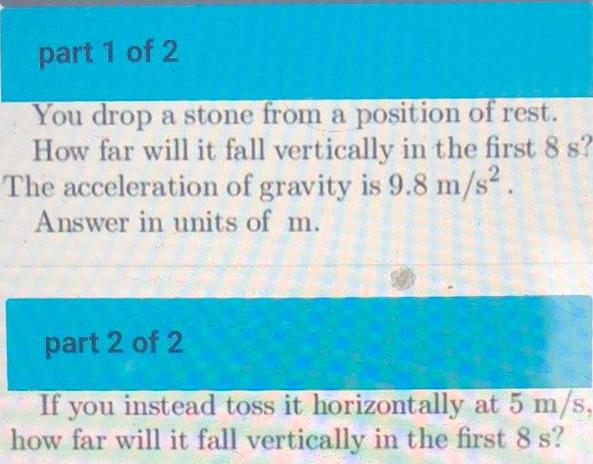Physics
Newton's law of motion
You drop a stone from a position of rest. How far will it fall vertically in the first 8 s? The acceleration of gravity is 9.8 m/s². Answer in units of m. If you instead toss it horizontally at 5 m/s, how far will it fall vertically in the first 8 s?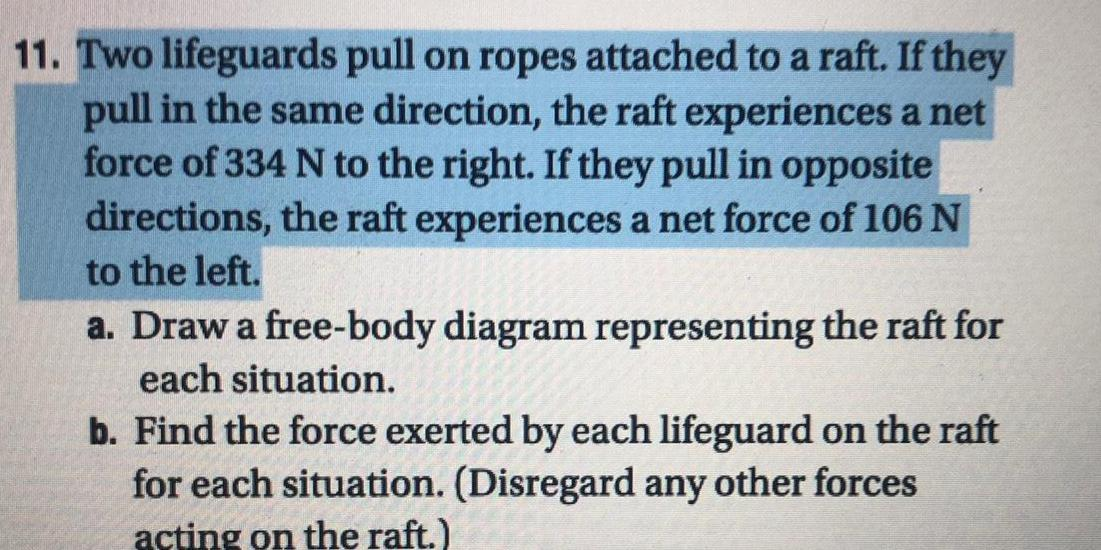Physics
Newton's law of motion
Two lifeguards pull on ropes attached to a raft. If they pull in the same direction, the raft experiences a net force of 334 N to the right. If they pull in opposite directions, the raft experiences a net force of 106 N to the left. a. Draw a free-body diagram representing the raft for each situation. b. Find the force exerted by each lifeguard on the raft for each situation. (Disregard any other forces acting on the raft.)